# 17.4: Nucleophilic Addition Reactions of Enolate Anions

$$\newcommand{\vecs}{\overset { \rightharpoonup} {\mathbf{#1}} }$$ $$\newcommand{\vecd}{\overset{-\!-\!\rightharpoonup}{\vphantom{a}\smash {#1}}}$$$$\newcommand{\id}{\mathrm{id}}$$ $$\newcommand{\Span}{\mathrm{span}}$$ $$\newcommand{\kernel}{\mathrm{null}\,}$$ $$\newcommand{\range}{\mathrm{range}\,}$$ $$\newcommand{\RealPart}{\mathrm{Re}}$$ $$\newcommand{\ImaginaryPart}{\mathrm{Im}}$$ $$\newcommand{\Argument}{\mathrm{Arg}}$$ $$\newcommand{\norm}{\| #1 \|}$$ $$\newcommand{\inner}{\langle #1, #2 \rangle}$$ $$\newcommand{\Span}{\mathrm{span}}$$ $$\newcommand{\id}{\mathrm{id}}$$ $$\newcommand{\Span}{\mathrm{span}}$$ $$\newcommand{\kernel}{\mathrm{null}\,}$$ $$\newcommand{\range}{\mathrm{range}\,}$$ $$\newcommand{\RealPart}{\mathrm{Re}}$$ $$\newcommand{\ImaginaryPart}{\mathrm{Im}}$$ $$\newcommand{\Argument}{\mathrm{Arg}}$$ $$\newcommand{\norm}{\| #1 \|}$$ $$\newcommand{\inner}{\langle #1, #2 \rangle}$$ $$\newcommand{\Span}{\mathrm{span}}$$

A most important property of enolate anions, at least as far as synthesis is concerned, is their excellent nucleophilicity, which enables them to add to double bonds and to participate in nucleophilic substitution. When the addition is to a carbonyl double bond, it is called an aldol addition (Equation 17-4). Additions of enolate anions to carbon-carbon double bonds usually are classified as Michael additions (Equation 17-5), and these are discussed in Sections 17-5B and 18-9D. The principles of $$S_\text{N}$$ nucleophilic reactions of enolate anions (Equation 17-6) will be considered in Section 17-4, and their synthetic applications in detail in Chapter 18.The products of aldol addition are $$\beta$$-hydroxy aldehydes (ald-ols) or $$\beta$$-hydroxyl ketones (ket-ols). A typical example is the reaction of ethanal with base and, if the conditions are reasonably mild, the product is 3-hydroxybutanal:The overall reaction corresponds to a dimerization of ethanal, that is, an addition of one ethanal molecule to another with formation of a new carbon-carbon bond. The synthetic value of the reaction lies in the fact that it can be used to build large molecules from smaller molecules (see Section 13-7).

Formation of the enolate anion, $$7$$, by removal of an $$\alpha$$ hydrogen by base is the first step in the aldol addition: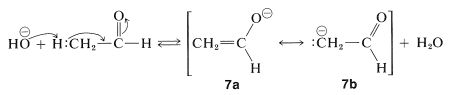The anion then adds to the carbonyl group of a second molecule of ethanal in a manner analogous to the addition of other nucleophiles to carbonyl groups (e.g., cyanide ion, Section 16-4A). The adduct so formed, $$8$$, rapidly adds a proton to the alkoxide oxygen to form the aldol, 3-hydroxybutanal. This last step regenerates the basic catalyst, $$\ce{OH}^\ominus$$:## Ambident Nature of Enolate Ions in Aldol Addition

The two possible valence-bond structures of the enolate anion, $$7a$$ and $$7b$$, show that the anion should act as an ambident nucleophile - a nucleophile with nucleophilic properties associated with both carbon and oxygen. The addition step in the aldol reaction therefore may be expected to take place in either of two ways: The anion could attack as a carbon nucleophile to form a carbon-carbon bond, $$8$$, leading ultimately to the aldol, $$9$$, or it might attack as an oxygen nucleophile to form a carbon-oxygen bond, thereby leading to the hemiacetal, $$10$$. By this reasoning, we should obtain a mixture of products $$9$$ and $$10$$. However, the aldol $$9$$ is the only one of these two possible products that can be isolated: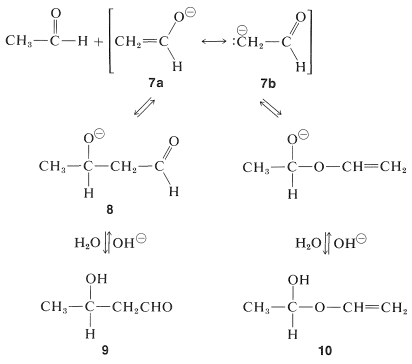Why is only one of these products formed? To understand this, you must recognize that aldol reactions are reversible and therefore are subject to equilibrium rather than kinetic control (Section 10-4A). Although the formation of $$10$$ is mechanistically reasonable, it is not reasonable on thermodynamic grounds. Indeed, while the overall $$\Delta H^0$$ (for the vapor) calculated from bond energies is $$-4 \: \text{kcal mol}^{-1}$$ for the formation of the aldol, it is $$+20.4 \: \text{kcal mol}^{-1}$$ for the formation of $$10$$.$$^2$$ Therefore, the reaction is overwhelmingly in favor of the aldol as the more stable of the two possible products.

## Position of the Equilibrium in Aldol Additions

The equilibrium constant is favorable for the aldol addition of ethanal, as in fact it is for most aldehydes. For ketones, however, the reaction is much less favorable. With 2-propanone (acetone) only a few percent of the addition product "diacetone alcohol", $$11$$ is present at equilibrium:The 2-propanone is boiled and the hot condensate from the reflux condenser flows back over solid barium hydroxide contained in the porous thimble and comes to equilibrium with the addition product $$11$$. The barium hydroxide is retained by the porous thimble and the liquid phase returns to the boiler where the 2-propanone, which boils $$110^\text{o}$$ below the temperature at which $$11$$ boils, is selectively vaporized and returns to the reaction zone to furnish more adduct.

The key step in aldol addition requires an electron-pair donor (nucleophile) and an electron-pair acceptor (electrophile). In the formation of 3-hydroxybutanal or $$11$$, both roles are played by one kind of molecule, but there is no reason why this should be a necessary condition for reaction. Many kinds of mixed aldol additions are possible.

Consider the combination of methanal and 2-propanone. Methanal cannot form an enolate anion because it has no $$\alpha$$ hydrogens. However, it is expected to be a particularly good electron-pair acceptor because of freedom from steric hindrance and the fact that it has an unusually weak carbonyl bond ($$166 \: \text{kcal}$$ compared to $$179 \: \text{kcal}$$ for 2-propanone). In contrast, 2-propanone forms an enolate anion easily but is relatively poor as the electrophile. Consequently the addition of 2-propanone to methanal should and does occur readily:The problem is not to get addition, but rather to keep it from going too far. Indeed, all six $$\alpha$$ hydrogens of 2-propanone can be replaced easily by $$\ce{-CH_2OH}$$ groups: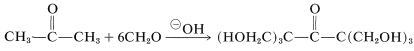A commercially important mixed addition involves ethanal and an excess of methanal in the presence of calcium hydroxide. Addition occurs three times and the resulting trihydroxymethylethanal (which has no $$\alpha$$ hydrogens) undergoes a "crossed Cannizzaro" reaction with more methanal to give a tetrahydroxy alcohol known as "pentaerythritol::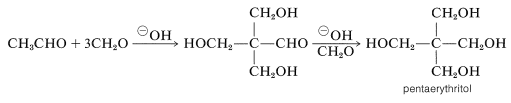Pentaerythritol is used widely in the preparation of surface coatings and in the formation of its tetranitrate ester, pentaerythrityl tetranitrate [PETN, $$\ce{C(CH_2ONO_2)_4}$$], which is an important high explosive.

## Dehydration of Aldol Addition Products

An important property of aldol addition products is the ease with which they eliminate water in the presence of either acids or bases. For example, when 3-hydroxybutanal is heated in the basic solution in which it is formed (by aldol addition of ethanal), 2-butenol results:The ease of dehydration compared with simple alcohols is related to the fact that the product is a conjugated alkenone. The stabilization energy of the conjugated system makes the equilibrium constant for dehydration especially favorable. In many cases the aldol adduct is only an intermediate in aldol reactions because it dehydrates more rapidly than it can be isolated. Such is most often the case when the dehydration product is a polyunsaturated conjugated aldehyde or ketone. 2-Propanone and bezenecarbaldehyde (benzaldehyde), for instance, give the unsaturated ketone $$12$$ in cold aqueous sodium hydroxide solution: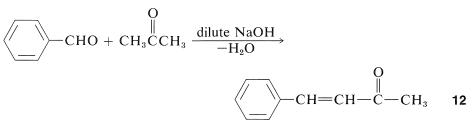Although the equilibrium for aldol addition may be unfavorable, when dehydration of the aldol product is rapid, $$\ce{C-C}$$ bond formation may be pushed to completion by conversion of the aldol to the $$\alpha$$,$$\beta$$-unsaturated ketone.

The mechanism of base-catalyzed dehydration of aldols involves formation of an enolate anion by removal of a proton from the $$\ce{C2}$$ or alpha carbon and subsequent elimination of the hydroxyl group as hydroxide ion:This last step is one of the rare examples in which the leaving group is $$\ce{OH}^\ominus$$. Generally, hydroxide is a poor leaving group in substitution ($$S_\text{N}1$$ or $$S_\text{N}2$$) or elimination ($$E1$$ or $$E2$$) reactions (see Section 8-7C).

Dehydration of aldols to $$\alpha$$,$$\beta$$-unsaturated carbonyl compounds usually is achieved best with acidic catalysts. An example is the dehydration of the aldol from 2-propanone to give 4-methyl-3-penten-2-one:If this reaction were attempted under basic conditions, extensive reversion of the aldol to 2-propanone would occur (see Section 17-3C). Under acidic conditions, however, the process is a straightforward proton transfer to oxygen followed by elimination of water and proton transfer from carbon:## The Use of Aldol Addition Reactions in Synthesis

Aldol reactions provide a valuable synthetic method for forming carbon-carbon bonds. They can be adapted to extend the length of a carbon chain, to form cyclic compounds, and to provide intermediates that can be transformed into more useful materials. An important feature of these intermediates is that functional groups useful for later reactions are located close to or on the carbons of the newly formed $$\ce{C-C}$$ bond. There is an almost bewildering number of variations on the aldol reaction and we shall not mention all of them. The main thing to recognize in all of these reactions is that the acceptor molecule always is a carbonyl compound, best an aldehyde, sometimes a ketone, even an ester (see Section 18-8E). The donor molecule is some type of carbanion; usually, but not always, an enolate anion. However, any substance that has a $$\ce{C-H}$$ acidity in the p$$K_\text{a}$$ range of 25 or less can be converted easily to a carbanion, which in principle may serve as the donor in aldol additions. Examples are listed in Table 17-1 and include not only aldehydes and ketones but esters, nitriles, and nitro compounds. The use of a nitroalkane in aldol addition is shown in the following sequence. The use of esters as the donor is discussed further in Section 18-8E.Cyclic products can be formed by aldol additions provided the donor carbanion and acceptor carbonyl are part of the same molecule. For example, consider how the synthesis of 3-methyl-2-cyclohexanone could be achieved from acyclic substances. The carbon-carbon bond formed in this process of aldol addition closes the ring and ultimately becomes the double bond in the conjugated system when the aldol product undergoes dehydration. Working backwards, we have the sequence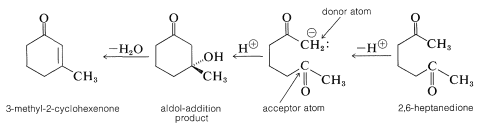and the starting material for the synthesis therefore is 2,6-heptanedione. Because $$\Delta G^0$$ for the formation of aldol products is not very favorable, cyclizations involving aldol reactions usually will not proceed to give strained carbocyclic rings.

The industrial importance of aldol reactions is in the synthesis of alcohols, especially 1-butanol and 2-ethyl-1-hexanol: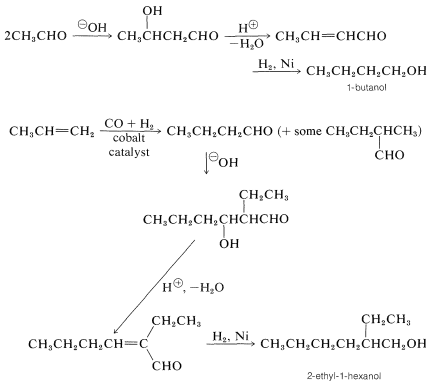Notice that the combination of hydroformylation (Section 16-9F), aldol addition, dehydration, and hydrogenation takes a simple alkene (propene) to an alcohol with more than twice as many carbons.

One of the reactions in the metabolism of carbohydrates by the glycolic pathway is a type of aldol addition. In this reaction $$D$$-fructose (as the 1,6-diphosphate ester) is formed from $$D$$-glyceraldehyde and 1,3-dihydroxypropanone (both as monophosphate esters). The process is readily reversible and is catalyzed by an enzyme known as aldolase: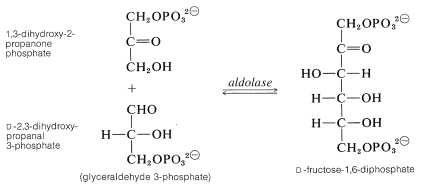It seems likely that this reaction could occur in quite the same way as in the laboratory aldol reactions discussed so far, because the enolate anion of the donor molecule (dihydroxypropanone) is not expected to be formed in significant amount at the pH of living cells. In fact, there is strong evidence that the enzyme behaves as an amino $$\left( \ce{ENH_2} \right)$$ compound and reacts with the carbonyl group of dihydroxypropanone to form an imine, analogous to the reactions described in Section 16-4C:This implies that the imine form of dihydroxypropanone is a key intermediate in the overall aldol-type addition.

How can the imine behave as the carbon donor in addition to the aldehyde carbonyl of glyceraldehyde 3-phosphate? It is unlikely to do so directly, but it can rearrange to an enamine which, as we will explain in Section 17-4B, can act as a carbon nucleophile: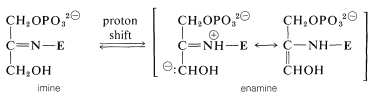Attack of the nucleophilic carbon of the enamine at the aldehyde carbonyl of glyceraldehyde 3-phosphate forms the aldol of the imine which, on hydrolysis, gives the aldol and regenerates the enzyme:By using the neutral enamine as the carbon nucleophile rather than an enolate anion, the biological system avoids the need for strongly basic reaction conditions in aldol addition.

$$^2$$This value probably is too large by $$3$$ to $$4 \: \text{kcal}$$, because resonance stabilization of alkoxyalkanes has been ignored in this calculation.